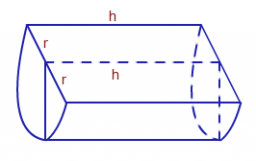# The circumference 3

The circumference of a cylindrical water tank is 62.8m. When it is 4/5 full of water, it holds 125.6hl. Find the depth of the tank.

h =  0.05 m

### Step-by-step explanation:Did you find an error or inaccuracy? Feel free to write us. Thank you!

Tips for related online calculators
Do you have a linear equation or system of equations and looking for its solution? Or do you have a quadratic equation?

#### Grade of the word problem:

We encourage you to watch this tutorial video on this math problem: